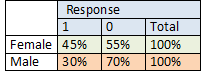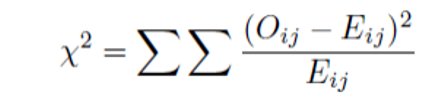Call Us @

(91) 96200 48623

## Blog: Test of Association for Categorical1

A T-test is often used when you want to compare whether two groups of data are significantly different from each other. We do this by comparing the means of the two different groups. For example, whether patients who received medication have higher T-cell counts compared to patients who didn't or whether students who attended special classes scored more that students who didn't. In all such cases, we work with continuous data like height, weight, salary, etc.

But what if we are dealing with categorical variables? Suppose we want to test if females are more likely to respond to a particular marketing campaign compared to males or in other words whether there is an association between gender and response variable. Since, both Gender and Response Variables are categorical, we have to use the Chi-square test which tests the association between two categorical variables.

As in the example below, 45% of females respond to the campaign while in males, only 30% are responders. The result could imply that there is some association between gender and response but is this association random or statistically significant? To ascertain this we will use the Chi-square test.### Chi-Square Test and Statistics

The Chi-square test is available in most of the statistical tools such as Python, R, and SAS  and gives you Chi-square statistics directly.  If you want to understand the calculations, please find an excel link that has step by step chi-square test statistics calculations.

Chi-square measures the difference between the observed frequencies and the expected frequencies which are calculated when there is no association between the variables, in other words, frequencies that are expected when the null hypothesis is true (hypothesis of no association). If the observed frequency equals expected frequency, there is no association between variables. Below is the formula for the Chi-Square statistic. Higher the chi-square value, the smaller the p-value and hence the higher chance of rejecting the null hypothesis.

∑((Observed freq-Expected Freq)^2/(Expected Freq))Here is an interesting question - Does a higher chi-square value indicate a stronger association between values? The answer is No. Chi-square does not test for the strength of the association between variables. Later in this article, we will see how to measure the strength of association.

Within the categorical variable, some variables are called ordinal variables. An ordinal variable is a variable that takes only a few distinct values but the level of the variable has order within the levels or the levels of a variable can be ordered in some meaningful way, like a response to a customer survey – extremely satisfied, somewhat satisfied, not satisfied at all.

Now, when we want to find an association between ordinal variables, a Mantel – Haenszel Chi-square test is a more powerful test for testing the ordinal association.

What we discussed earlier is called Pearson Chi-Square Test. Please note that the Mantel – Haenszel Chi-square test can be used only if both variables are ordinal. Interpretation of this statistic is similar to Pearson’s Chi-square that is higher the value, the smaller the p-value and hence the higher chance of rejecting the null hypothesis.

Measuring the strength of Association - Cramer’s V statistic and Spearman correlation

This brings us to our last topic of today’s discussion. Cramer’s V statistic is used to measure the strength of association between categorical variables. Values closer to 1 show strong association while values closer to 0 shows weak or no association. Another important aspect of Cramer’s V statistic is that it is not impacted by sample size as compared to the Chi-square statistic which yields a higher value for a bigger sample size.

For ordinal variables, a Spearman correlation statistic can be used to test the strength of association. Similar to Cramer’s V statistic, values closer to 1/-1 indicate strong positive or negative association respectively while values closer to 0 indicate a weaker association. Values are not impacted by sample size as in the case of Cramer’s V.

### Tags

• SOCIAL SHARE :